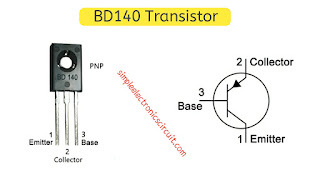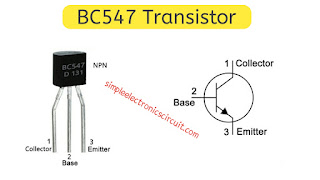# New 1.5 volt to 220 volt inverter circuit diagram 2020

New 1.5 volt to 220-volt inverter circuit diagram 2020New 1.5 volt to 220 volt inverter circuit diagram 2020
Hello, guys, I will explain this circuit this is the circuit of 1.5 volts to put on the walls in order many of the people ask you about this sector so I am making this video here you can see here is this is the switching transitions IQ this will be switched to generate the square wave or square waves here you can see the residual a capacitor this is this capacitor is for tuning purpose and if you increase the capacitance.

the frequency will reduce frequency if you decrease the capacitor frequency will increase and here you can see the counselor transformer and this way I recommend you to use the transformer of 3 amps to 5 amp transformer and when you give the input here destroy this transistor stresses witching and generate the square wave here this craving goes to the transformer and you can hear get the AC generation here and this is the 1.5volts input and when the input is given and this capacitance cross this charging-discharging the rate of thing if you increase.

the capacitance the discharging on charging rate will be less so there you can get the square waves with lesser frequency and if you decrease the size of the capacitance here and you then you will get the frequency with the higher frequency because the charging and discharging time will be very less and that this is alternating the arrays square pulse goes to this transformer and due to the mutual induction you can get the higher voltage output I recommend you to use the six volts.

to the divorce three am Sofa answer for a transformer and this is as one of these and this is the NPN transistor and this is the PNP transistor the switching rates wrote on this only chemistry and this is the main importance of this main the role played by the components are this one this capacitor and the transformer this is the capacity to set the frequency and transform of generally higher voltage.

how to easily make a 1.5-volt inverter. Let's see how easy it is to make a 1.5-volt inverter. First of all, a transistor bd140 was taken And another transistor bc547 is taken two transistors The base of a transistor bd140 is connected to the bc547 collector pin of the transistor and the bc147 transistor is connected to the bd140 transistor pin A 30k register is attached to the base pin of it. A 100nf capacitor is taken. A pin of the capacitor is attached to the bc547 transistor base. And another pin bd140 is connected to the emitter pin of the transistor,

Our circuit is ready. Only one 6,0 transformer needs to be added so we now have the transformer with the circuit we makeLet's add Transformer 6,0 to any one of its bd140 transistors And connected to the emitter and again another transformer on the other end was connected to its bc547 transistor and our 1.5-volt inverter circuit was fully formed.

Battery Supply: In order for our 1.5-volt inverter circuit to work, we need to supply a 1.5-volt battery in the circuit and the collector pin of the battery (+) bd140 transistor pin It is associated with. And the battery (-) is connected to the emitter pin of its bc547 transistor.

Working: After supplying our 1.5-volt inverter circuit, 220-volt ac supply is coming out at the output of the transformer.

## Components:

1) 30K Ohm resistor
2) 0.1uF capacitor
3) Transformer (6v:220v)
4) AABattery Case
5) Switch
6) Perforated PCB
7) BC 547 transistor
8) BD140 Transistor with heat sink

### Circuit Diagram:New 1.5 volt to 220 volt inverter circuit diagram 2020

# BD140 Transistor PinoutNew 1.5 volt to 220 volt inverter circuit diagram 2020

# BD140 Transistor Features

• Transistor Type: PNP
• Max Collector Current(IC): -1.5A
• Max Collector-Emitter Voltage (VCE): –80V
• Max Collector-Base Voltage (VCB): –80V
• Max Emitter-Base Voltage (VEBO): –5V
• Max Collector Dissipation (Pc): 12.5 Watt
• Max Transition Frequency (fT): 190 MHz
• Minimum & Maximum DC Current Gain (hFE): 25 – 250

### BC547 Transistor PinoutNew 1.5 volt to 220 volt inverter circuit diagram 2020

### BC547 Transistor Features

• Bi-Polar NPN Transistor
• DC Current Gain (hFE) is 800 maximum
• Continuous Collector current (IC) is 100mA
• Emitter Base Voltage (VBE) is 6V
• Base Current(IB) is 5mA maximum

### BC547 Transistor Explained

Transistor bc547 is a BJT NPN transistor, The bc547 transistor is used in electronics educational projects. And is used in projects other than this one, And this transistor is the maximum output 100MA.